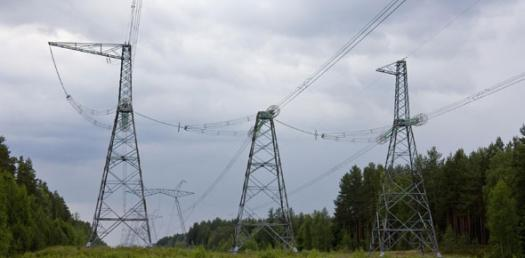# Electricity And Ohms Law Questions

9 Questions | Attempts: 1318SettingsMaterial on Ohm's Law, Voltage, Current, and Resistance.

• 1.
What is the unit for voltage?
• A.

Volts

• B.

Amperes

• C.

Ohms

• D.

Joules

• 2.
What is the unit for current?
• A.

Volts

• B.

Amperes

• C.

Ohms

• D.

Joules

• 3.
What is the unit for resistance?
• A.

Volts

• B.

Amperes

• C.

Ohms

• D.

Joules

• 4.
Which of these is the equation for Ohm's Law?
• A.

W = Fd

• B.

V = IR

• C.

R = IV

• D.

PE = mgh

• 5.
A certain steam iron carries a current of 6.4 A when connected to a 120 V source.  What is the resistance of the steam iron?
• A.

1.875 Ω

• B.

0.05 Ω

• C.

768 Ω

• D.

18.75 Ω

• 6.
The resistance of a hotplate is 48 Ω .  How much current does the plate carry when connected to a 120-V source?
• A.

120 A

• B.

2.5 A

• C.

5760 A

• D.

0.4 A

• 7.
A typical size-D battery has about how many volts?
• A.

0.05 V

• B.

100 V

• C.

1.5 V

• D.

9 V

• 8.
Voltage is a measurement of what?
• A.

Radiant energy of a lightbulb in a circuit

• B.

Kinectic energy of a motor in a circuit

• C.

Potential energy stored in a battery

• D.

The flow of electrons

• 9.
Electrical Power is measured in :
• A.

Watts

• B.

Amperes

• C.

Volts

• D.

Meters

## Related TopicsBack to top
×

Wait!
Here's an interesting quiz for you.